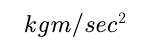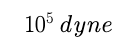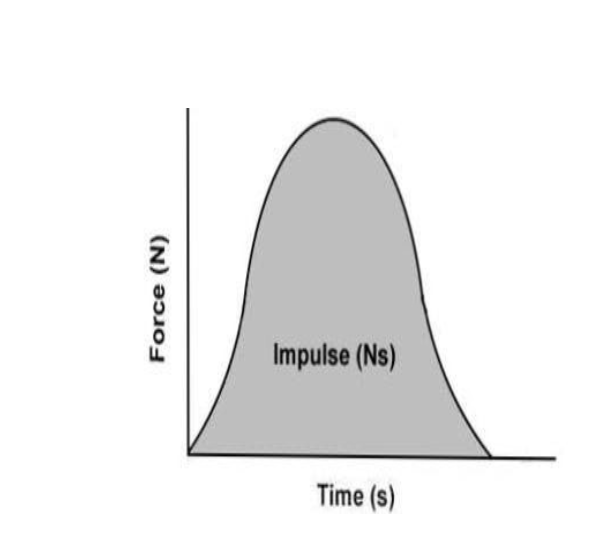# Laws Of Motion , Law of Inertia, Impulse and Impulsive Force

Laws Of Motion

Inertia : It is inherent characteristic due to change its condition of motion.

There are three types of inertia. It is scalar quantity

A: Inertia of rest : The opposition of body due to change its state of rest.

B: Inertia of motion: The opposition of body due to change its state of motion.

C: Inertia of direction: It is inability of a body to change its direction of motion.

Important point -

Mass is the measurement of inertia.

Inertia is depend on mass.

Force:

Is push or pull. It changes a condition of motion.

It is a vector quantity.

System of particles:

It is a group of particles having same velocity and fixed distance between them.

Momentum:

It is characteristic of a moving object.

It denoted by P.

P=mv

It is vector quantity

Si unit of momentum is kgm/sec^2.

Newton’s First Law ( Law of Inertia) :

An object stay at rest or motion continue unless an external force is applied on it.

Important points:

It defines inertia, force and mechanical equilibrium, Net acceleration of object is zero.

First law gives definition of force.

Newton Second Law:

Rate of change of momentum is directly proportionate to the net external force is applied on object.

Second law gives quantity of force

F∝ dp/dt

F∝ d(mv)/dt

F∝ mdv/dt.              (dv/dt=a)

F∝ ma –eq (i)

F= kma , where k is constant.

If F=1 , m=1 , a=1 then k=1

Putting value of k in equation (i)

Then F= ma.

Si unit of force isCGS unit = dyne.

One newton =Force is a vector quantity.

Dimension= MLT^−2.

Second Law : Impulse and Impulsive Force

A large number of force can create in very small interval of time.

Impulse = Force × (final time – initial time)
Impulse = Force × Δt
I = F × Δ

Derivation of the Formula

I = refers to the impulse
F = refers to the force of the object
Δt = refers to the change in timeSince the impulse is a measure of how much the momentum changes as a result of a force acting on it for a period of time. Moreover, an alternative formula for impulse is

Impulse = Δp  = p final – p initial

Impulse (I) = F x t = m(v-u).

Si unit= Nsec. And kgm/sec.

Dimension = MLT^-1

Post By : Faiz Ahmad Faiz 18 Feb, 2020 2734 views Physics Laws of Motion and Friction